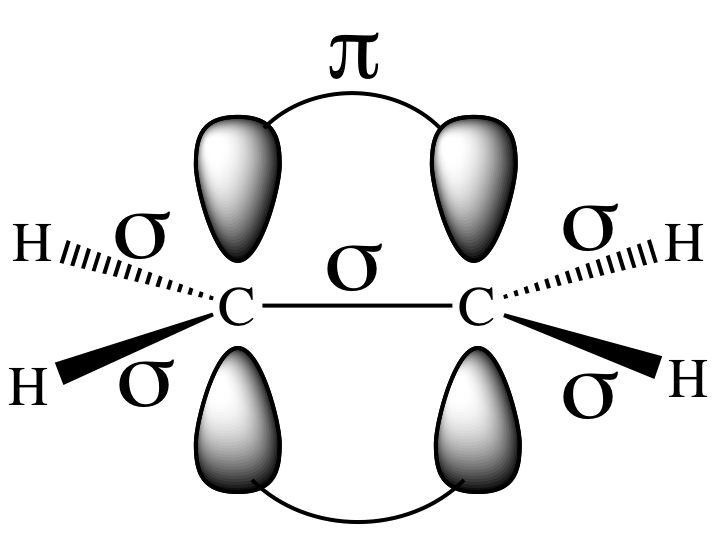What does a pi bond consist of?

This diagram shows ethene (an alkene). Each carbon has three $s {p}^{2}$ hybridised orbitals that form three sigma $\left(\sigma\right)$ bonds and the remaining p orbital forms the pi $\left(\pi\right)$ bond.